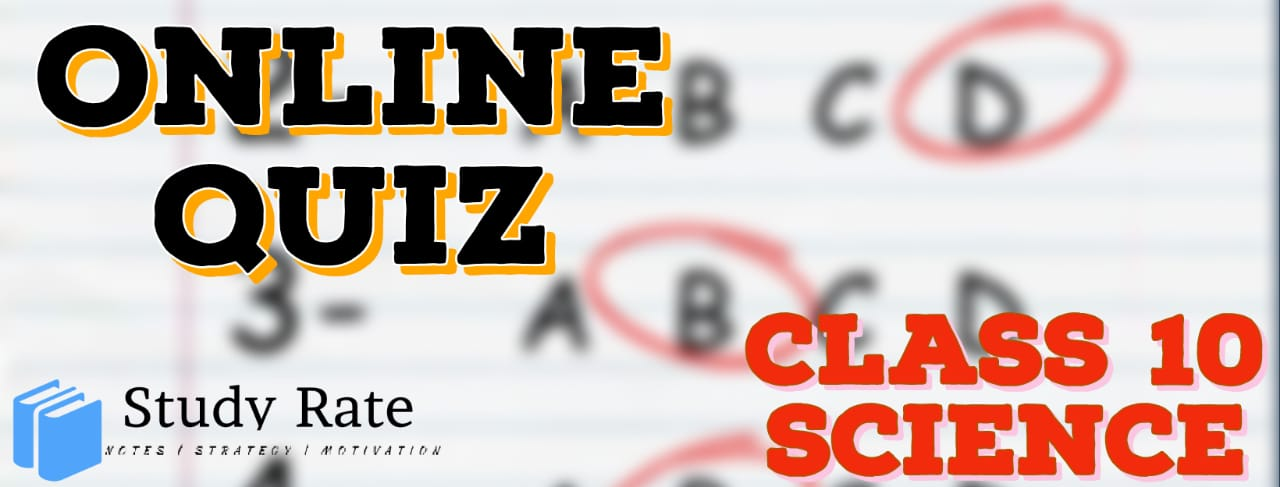## CBSE Class 10 Science Periodic Classification of Elements MCQ Quiz with Answers

Free PDF Download of CBSE Class 10 Science Chapter 5 Periodic Classification of Elements Multiple Choice Questions with Answers. MCQ Questions for Class 10 Science with Answers were Prepared Based on Latest Exam Pattern. Students can solve NCERT Class 10 Science Periodic Classification of Elements Multiple Choice Questions with Answers to know their preparation level.

Periodic Classification of Elements Class 10 Science MCQ Quiz Online

1. How many periods and groups are present in the periodic table?

2. Which of the following forms the basis of the modern periodic table?

3. An element M is in group 13th of the periodic table, the formula for its oxide is

4. An element X belongs to the 3rd period and 1st group of the periodic table. What is the number of valence electrons in its atom?

5. Which of the following does not decrease while moving down the group of the periodic table?

6. Which of the following is the atomic number of an element that forms basic oxide?

7. The elements A, B and C belong to group 2, 14 and 16 respectively, of the periodic table. Which of the two elements will form covalent bonds?

8. Which group elements are called transition metals?

9. Element X forms a chloride with the formula XCl2, which is a solid with a high melting point. X would most likely be in the same group of the Periodic Table as

10. Which of the following is the most reactive element of the group 17?